## Example Questions

### Example Question #1 : How To Divide Square Roots

What is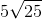divided by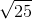?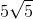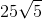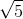Explanation:

Upon dividing by, thein the numerator cancels out, and the only number remaining is: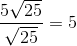### Example Question #2 : How To Divide Square Roots

Simplify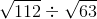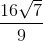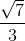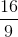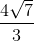Explanation:

Find the greatest factor that is a perfect square for 112 and 63. For 112, the factors are 16 and 7, thus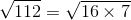. For 63, the factors are 9 and 7, thus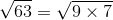. Simplifying these terms will give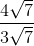. Cancel out the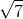results in.

### Example Question #3 : How To Divide Square Roots

Simplify the following expression: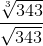Cannot be simplified any further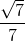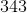Explanation:

Although it may seem as though we cannot do anything to this expression due to our numerator and denominator having different indices, there is in fact some real simplifying to be done here.

To begin, our numerator can be evaluated, because 343 is in fact a perfect cube: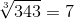This fact helps us out with our denominator as well. Our original equation becomes the following: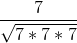Then, we can pull out two of the sevens on the bottom and cancel them like so: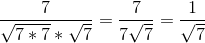We may seem to be done, but if you look, you will not see our solution among the answer choices. That is because we need to rationalize the denominator. We should never have a square root in our denominator. To remedy this, we do a fairly simple move that won't change the value of our fraction, but just the form it is in: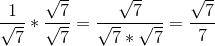Now we'll have no issue finding our answer choice!

### Example Question #4 : How To Divide Square Roots

Simplify: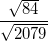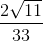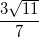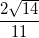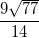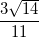Explanation:

Division of square roots is easy, since you can combine the roots and treat it like any other fraction.  Thus, you can convert our fraction as follows: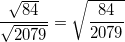Next, you begin to reduce the fraction: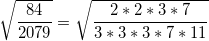This reduces to: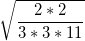Now, break this apart again into: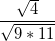, which is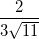Finish by rationalizing the denominator: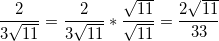### Example Question #5 : How To Divide Square Roots

Simplify: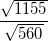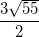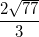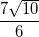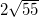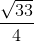Explanation:

Division of square roots is easy, since you can combine the roots and treat it like any other fraction. Thus, you can convert our fraction as follows: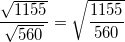Next, you begin to reduce the fraction: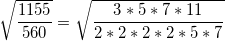This reduces to: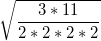Now, break this apart again into: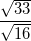, which is